# NEWTON.pptx

18 de May de 2023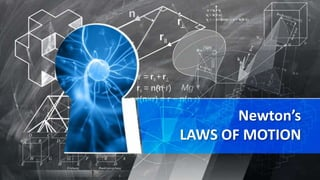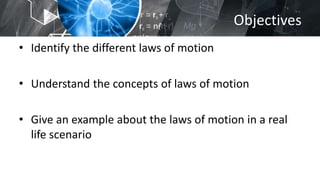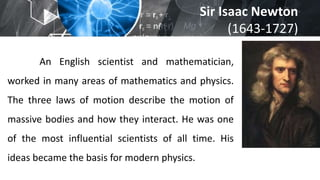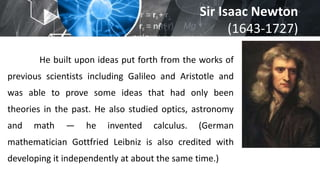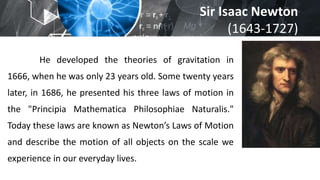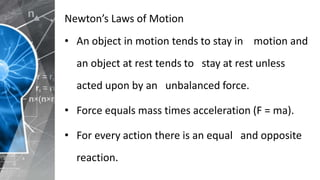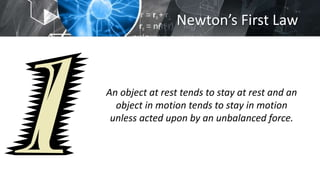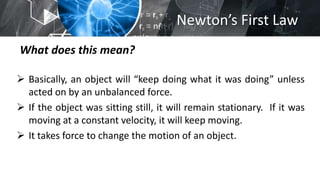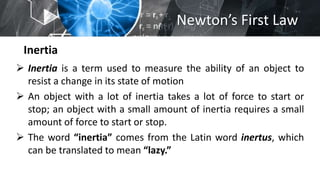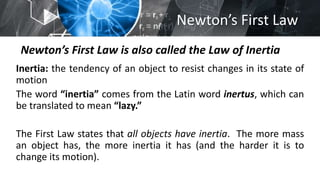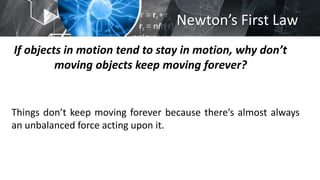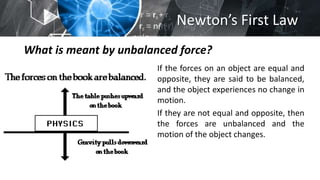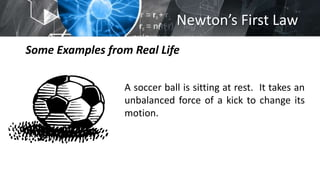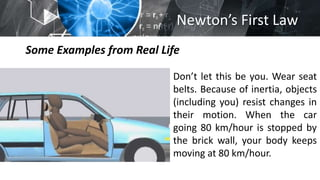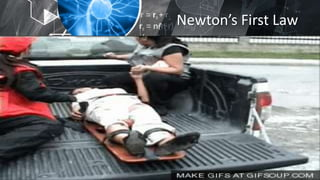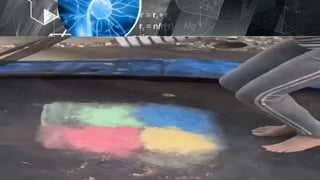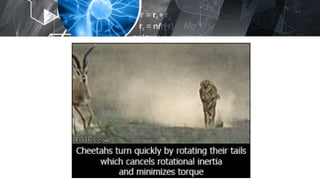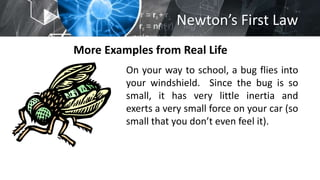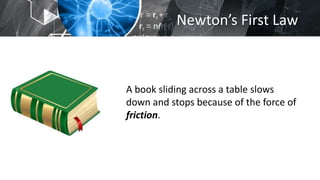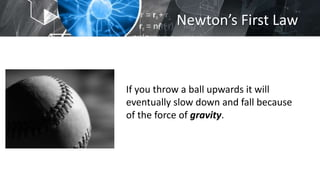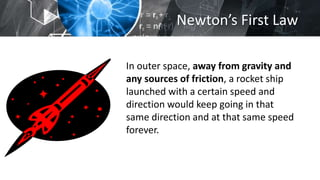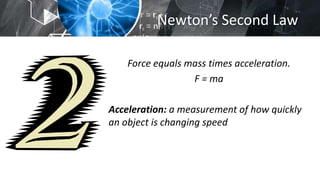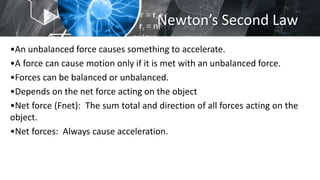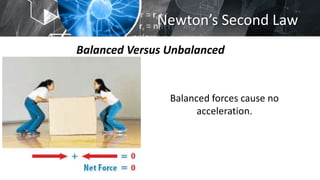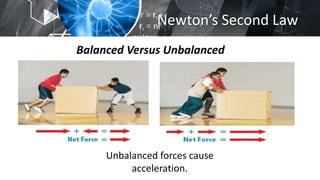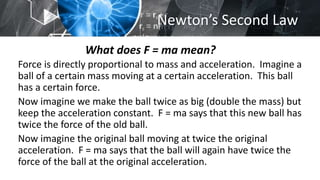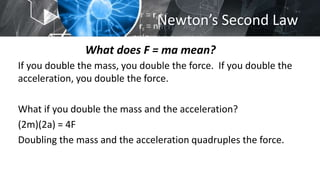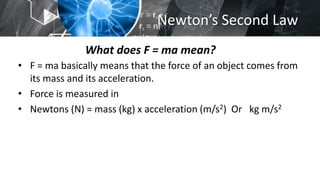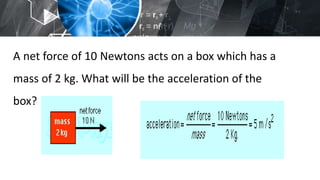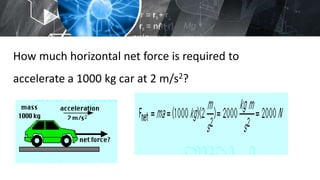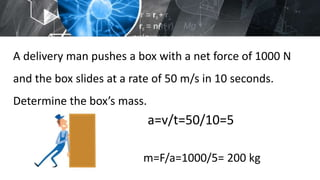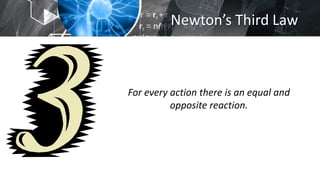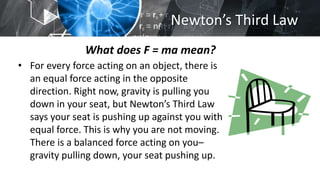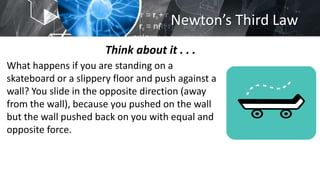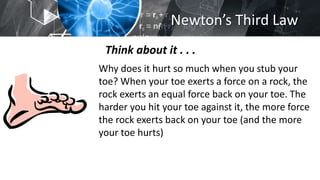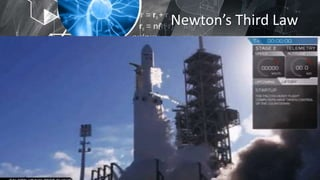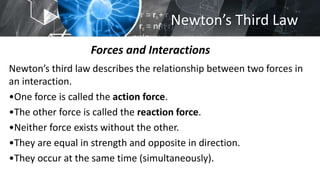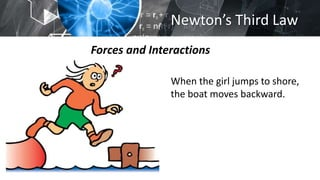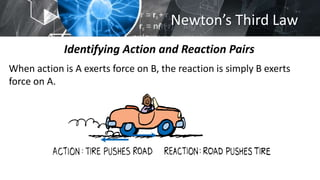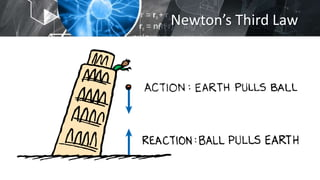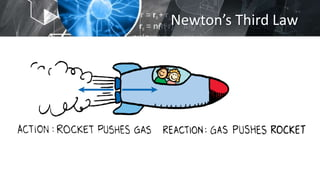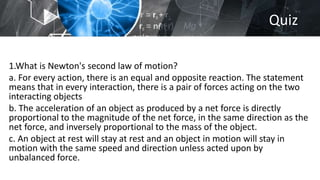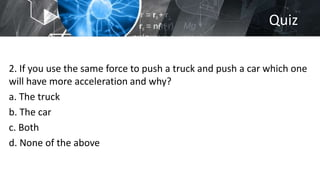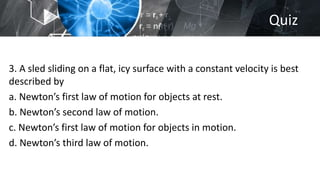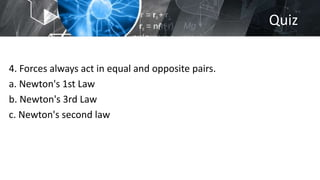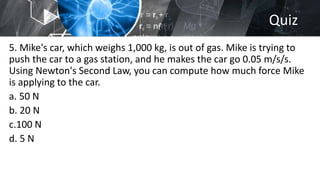1 de 48

### NEWTON.pptx

• 2. Objectives • Identify the different laws of motion • Understand the concepts of laws of motion • Give an example about the laws of motion in a real life scenario
• 3. Sir Isaac Newton (1643-1727) An English scientist and mathematician, worked in many areas of mathematics and physics. The three laws of motion describe the motion of massive bodies and how they interact. He was one of the most influential scientists of all time. His ideas became the basis for modern physics.
• 4. Sir Isaac Newton (1643-1727) He built upon ideas put forth from the works of previous scientists including Galileo and Aristotle and was able to prove some ideas that had only been theories in the past. He also studied optics, astronomy and math — he invented calculus. (German mathematician Gottfried Leibniz is also credited with developing it independently at about the same time.)
• 5. Sir Isaac Newton (1643-1727) He developed the theories of gravitation in 1666, when he was only 23 years old. Some twenty years later, in 1686, he presented his three laws of motion in the "Principia Mathematica Philosophiae Naturalis." Today these laws are known as Newton’s Laws of Motion and describe the motion of all objects on the scale we experience in our everyday lives.
• 6. Newton’s Laws of Motion • An object in motion tends to stay in motion and an object at rest tends to stay at rest unless acted upon by an unbalanced force. • Force equals mass times acceleration (F = ma). • For every action there is an equal and opposite reaction.
• 7. Newton’s First Law An object at rest tends to stay at rest and an object in motion tends to stay in motion unless acted upon by an unbalanced force.
• 8. Newton’s First Law What does this mean?  Basically, an object will “keep doing what it was doing” unless acted on by an unbalanced force.  If the object was sitting still, it will remain stationary. If it was moving at a constant velocity, it will keep moving.  It takes force to change the motion of an object.
• 9. Newton’s First Law Inertia  Inertia is a term used to measure the ability of an object to resist a change in its state of motion  An object with a lot of inertia takes a lot of force to start or stop; an object with a small amount of inertia requires a small amount of force to start or stop.  The word “inertia” comes from the Latin word inertus, which can be translated to mean “lazy.”
• 10. Newton’s First Law Newton’s First Law is also called the Law of Inertia Inertia: the tendency of an object to resist changes in its state of motion The word “inertia” comes from the Latin word inertus, which can be translated to mean “lazy.” The First Law states that all objects have inertia. The more mass an object has, the more inertia it has (and the harder it is to change its motion).
• 11. Newton’s First Law If objects in motion tend to stay in motion, why don’t moving objects keep moving forever? Things don’t keep moving forever because there’s almost always an unbalanced force acting upon it.
• 12. Newton’s First Law What is meant by unbalanced force? If the forces on an object are equal and opposite, they are said to be balanced, and the object experiences no change in motion. If they are not equal and opposite, then the forces are unbalanced and the motion of the object changes.
• 13. Newton’s First Law Some Examples from Real Life A soccer ball is sitting at rest. It takes an unbalanced force of a kick to change its motion.
• 14. Newton’s First Law Some Examples from Real Life Don’t let this be you. Wear seat belts. Because of inertia, objects (including you) resist changes in their motion. When the car going 80 km/hour is stopped by the brick wall, your body keeps moving at 80 km/hour.
• 18. Newton’s First Law More Examples from Real Life On your way to school, a bug flies into your windshield. Since the bug is so small, it has very little inertia and exerts a very small force on your car (so small that you don’t even feel it).
• 19. Newton’s First Law A book sliding across a table slows down and stops because of the force of friction.
• 20. Newton’s First Law If you throw a ball upwards it will eventually slow down and fall because of the force of gravity.
• 21. Newton’s First Law In outer space, away from gravity and any sources of friction, a rocket ship launched with a certain speed and direction would keep going in that same direction and at that same speed forever.
• 22. Newton’s Second Law Force equals mass times acceleration. F = ma Acceleration: a measurement of how quickly an object is changing speed
• 23. Newton’s Second Law Force equals mass times acceleration. F = ma Acceleration: a measurement of how quickly an object is changing speed
• 24. Newton’s Second Law •An unbalanced force causes something to accelerate. •A force can cause motion only if it is met with an unbalanced force. •Forces can be balanced or unbalanced. •Depends on the net force acting on the object •Net force (Fnet): The sum total and direction of all forces acting on the object. •Net forces: Always cause acceleration.
• 25. Newton’s Second Law Balanced Versus Unbalanced Balanced forces cause no acceleration.
• 26. Newton’s Second Law Balanced Versus Unbalanced Unbalanced forces cause acceleration.
• 27. Newton’s Second Law What does F = ma mean? Force is directly proportional to mass and acceleration. Imagine a ball of a certain mass moving at a certain acceleration. This ball has a certain force. Now imagine we make the ball twice as big (double the mass) but keep the acceleration constant. F = ma says that this new ball has twice the force of the old ball. Now imagine the original ball moving at twice the original acceleration. F = ma says that the ball will again have twice the force of the ball at the original acceleration.
• 28. Newton’s Second Law What does F = ma mean? If you double the mass, you double the force. If you double the acceleration, you double the force. What if you double the mass and the acceleration? (2m)(2a) = 4F Doubling the mass and the acceleration quadruples the force.
• 29. Newton’s Second Law What does F = ma mean? • F = ma basically means that the force of an object comes from its mass and its acceleration. • Force is measured in • Newtons (N) = mass (kg) x acceleration (m/s2) Or kg m/s2
• 31. A net force of 10 Newtons acts on a box which has a mass of 2 kg. What will be the acceleration of the box?
• 32. How much horizontal net force is required to accelerate a 1000 kg car at 2 m/s2?
• 33. A delivery man pushes a box with a net force of 1000 N and the box slides at a rate of 50 m/s in 10 seconds. Determine the box’s mass. a=v/t=50/10=5 m=F/a=1000/5= 200 kg
• 34. Newton’s Third Law For every action there is an equal and opposite reaction.
• 35. Newton’s Third Law What does F = ma mean? • For every force acting on an object, there is an equal force acting in the opposite direction. Right now, gravity is pulling you down in your seat, but Newton’s Third Law says your seat is pushing up against you with equal force. This is why you are not moving. There is a balanced force acting on you– gravity pulling down, your seat pushing up.
• 36. Newton’s Third Law Think about it . . . What happens if you are standing on a skateboard or a slippery floor and push against a wall? You slide in the opposite direction (away from the wall), because you pushed on the wall but the wall pushed back on you with equal and opposite force.
• 37. Newton’s Third Law Think about it . . . Why does it hurt so much when you stub your toe? When your toe exerts a force on a rock, the rock exerts an equal force back on your toe. The harder you hit your toe against it, the more force the rock exerts back on your toe (and the more your toe hurts)
• 38. Newton’s Third Law Think about it . . .
• 39. Newton’s Third Law Forces and Interactions Newton’s third law describes the relationship between two forces in an interaction. •One force is called the action force. •The other force is called the reaction force. •Neither force exists without the other. •They are equal in strength and opposite in direction. •They occur at the same time (simultaneously).
• 40. Newton’s Third Law Forces and Interactions When the girl jumps to shore, the boat moves backward.
• 41. Newton’s Third Law Identifying Action and Reaction Pairs When action is A exerts force on B, the reaction is simply B exerts force on A.
• 44. Quiz 1.What is Newton's second law of motion? a. For every action, there is an equal and opposite reaction. The statement means that in every interaction, there is a pair of forces acting on the two interacting objects b. The acceleration of an object as produced by a net force is directly proportional to the magnitude of the net force, in the same direction as the net force, and inversely proportional to the mass of the object. c. An object at rest will stay at rest and an object in motion will stay in motion with the same speed and direction unless acted upon by unbalanced force.
• 45. Quiz 2. If you use the same force to push a truck and push a car which one will have more acceleration and why? a. The truck b. The car c. Both d. None of the above
• 46. Quiz 3. A sled sliding on a flat, icy surface with a constant velocity is best described by a. Newton’s first law of motion for objects at rest. b. Newton’s second law of motion. c. Newton’s first law of motion for objects in motion. d. Newton’s third law of motion.
• 47. Quiz 4. Forces always act in equal and opposite pairs. a. Newton's 1st Law b. Newton's 3rd Law c. Newton's second law
• 48. Quiz 5. Mike's car, which weighs 1,000 kg, is out of gas. Mike is trying to push the car to a gas station, and he makes the car go 0.05 m/s/s. Using Newton's Second Law, you can compute how much force Mike is applying to the car. a. 50 N b. 20 N c.100 N d. 5 N